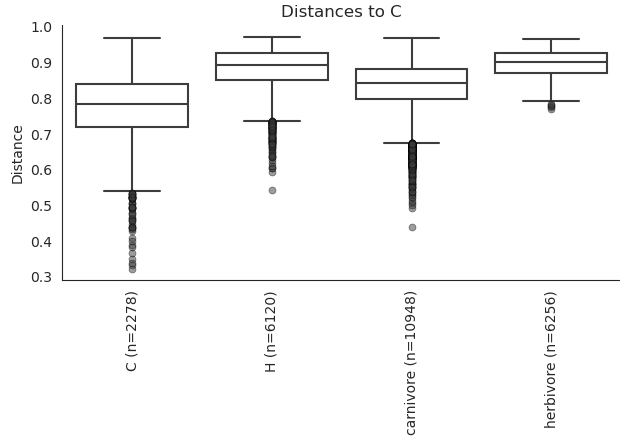comparing F values from PERMANOVA for beta diversity

Hi Qiime people,

I know that normally the point of beta diversity tests is to tell you that two groups are significantly dissimilar (in around about hypothesis rejection way) but I have the issue where I want to say two groups are significantly SIMILAR. I have a PERMANOVA result pasted below where two groups seem to be more similar to each other (C and carnivore) than the other two groups based on the distances and the F values. On the PCOA plot it is also apparent. However, I want to quantitively state that they are. The two options I see are ranking the distance values given by the test to show that two groups have significantly lower distances than the rest OR to compare the F values and show that two groups have significantly lower pseudo F values when comparing one to the other (basically comparing effect sizes).

My question is, do any of these two methods work to show significant "similarity" or is there a better test out there?

Thank you!Hey Sam,

I'm not a card-carrying statistician, but I found this helpful and thought I would share:
‘‘Not Statistically Different’’ Does Not Necessarily Mean ‘‘the Same’’: The Important but Underappreciated Distinction Between Difference and Equivalence Studies (PDF)

From that article:

For the equivalence trial, the null hypothesis is that |Mean1 – Mean2| >= d, where d is
a prespecified threshold equal to the largest difference that is still considered to be ‘‘clinically meaningless.’’ Deciding on this threshold (d) is a difficult and critically important aspect of the equivalence design. It serves as the operational definition of ‘‘the same as.’’ The alternative hypothesis is that |Mean1 – Mean| < d, or that the confidence interval for the difference between the means falls within the prespecified threshold.

I'm interesting to see if we can get a statistician to 'qiime-in' on this question.1 Like

Thanks for the input. Most of my research is constantly trying to show differences so that article is very relevant for my efforts in this case. However, I guess in my case my groups are all "significantly different" and I want to show that two of them are less "significantly different" than the rest. Or more simply put, how can I show that they are closer together on the darn PCOA plot in a quantitative way ? Does comparing the F values from the Permanova test suffice?
Thanks,
Sam

Oh wait, I've done this, lol (Colin & friends 2019)

In my study, the groups were samping day, and I wanted to show that the starting and ending days were self-similar, but the largest difference was between starting and ending days.

So I graphed the distance matrix as a heatmap. Brighter colors show greater differences, and you see that the start-to-end is much larger than start-to-start or end-to-end, because it'sHere, I'm reporting a vegan::adonis() test, but you could also do a t-test comparing the means of start-to-end vs start-to-start to show their difference. This is pretty much what diversity beta-group-significance does!Sure, you can think of the pseudo-F value as measure of effect-size. link

I'm basically trying to reframe my question so I can compare means and use a normal stat test.